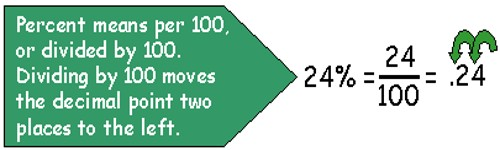Determining Percentage

Percent means “for every 100” or “out of 100.” The (%) symbol as a quick way to write a fraction with a denominator of 100. As an example, instead of saying “it rained 14 days out of every 100,” we say “it rained 14% of the time.”

Example: 68 is what percent of 87?

Divide the first number by the second (e.g. 68 ÷ 87 = 0.7816)

Multiply the answer by 100 (Move decimal point two places to the right) (e.g. 0.7816*100 = 78.16)

Round to the desired precision (e.g. 78.16 rounded to the nearest whole number = 78)

Follow the answer with the % sign (e.g. 68 is 78% of 87)

To determine the percent of a number do the following steps:

• Multiply the number by the percent (e.g. 87 * 68 = 5916)
• Divide the answer by 100 (Move decimal point two places to the left) (e.g. 5916/100 = 59.16)
• Round to the desired precision (e.g. 59.16 rounded to the nearest whole number = 59)To calculate the percentage increase:

First: work out the difference (increase) between the two numbers you are comparing.

Increase = New Number – Original Number

Then:  divide the increase by the original number and multiply the answer by 100.

% increase = Increase ÷ Original Number × 100

If your answer is a negative number then this is a percentage decrease.

To calculate percentage decrease:

First: work out the difference (decrease) between the two numbers you are comparing.

Decrease = Original Number – New Number

Then: divide the decrease by the original number and multiply the answer by 100.

% Decrease = Decrease ÷ Original Number × 100

If your answer is a negative number then this is a percentage increase.

If you wish to calculate the percentage increase or decrease of several numbers then we recommend using the first formula. Positive values indicate a percentage increase whereas negative values indicate percentage decrease.

Information Source: